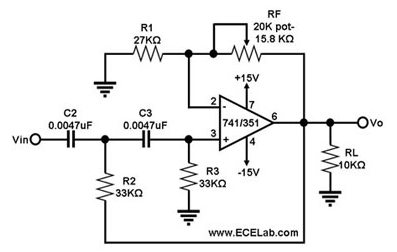# 巴特沃斯高通滤波器

## 巴特沃斯高通滤波器

### 二阶巴特沃斯高通滤波器电路图As the frequency of the input signal goes lower than fc, the gain of the first-order Butterworth high-pass filter in Figure 1 decreases at a rate of -20 dB/decade. If one desires a better high-pass frequency response than this, the second-order Butterworth high-pass filter in Figure 2 can be used. This circuit exhibits a -40 dB/decade roll-off at f<fc, wherein fc = 1/{2π x sqrt(R2R3C2C3)}. Also, for this circuit, the magnitude of Vo/Vin = (1+RF/R1)/(sqrt(1+(fc/f)4)).

### 高通滤波器